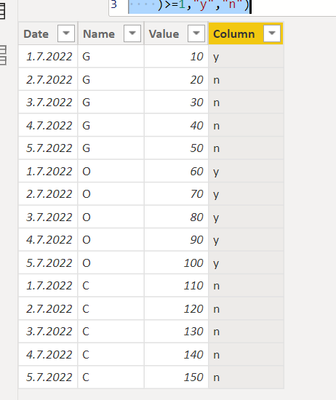cancel
Showing results for
Did you mean:New Member

## Retrieving information rom within a field.

I have 5 different columns that I need to extract infomation from and put the results in one column.  The following is what I have written to accomplish this, but it is not working.  Can anyone tell me what I am doing wrong?

AFR = IF(ISNUMBER(SEARCH("AFR",[Title])),"Y",IF(ISNUMBER(SEARCH("AFR”,[Finance Approve?])),"Y",IF(ISNUMBER(SEARCH("AFR",[Notes])),"Y",IF(ISNUMBER(SEARCH("AFR",[Notes_ 1])),"Y",IF(ISNUMBER(SEARCH("AFR",[Declined Reason])),"Y",IF(ISNUMBER(SEARCH("AFR",[Construction Request Type])),"Y","N"))))))

Any help woud be great.  Thanks.
1 ACCEPTED SOLUTIONSuper User

Hi,

I guess you are trying to check if one of these 5 columns include "AFR"?

Try using this kind of logic:

Dax:

Column = IF(
countrows(FILTER('Table',OR(EARLIER('Table'[Value])=10,EARLIER('Table'[Name])="o"))
)>=1,"y","n")

End result:This Dax tests if a [Value] in a table contains value 10 or [name] = "O". By using this logic you could create something like

Column = IF(
countrows(FILTER('Table',OR(
EARLIER('Table'[C1])="AFR",
EARLIER('Table'[C2])="AFR"),
EARLIER('Table'[C3])="AFR",
EARLIER('Table'[C4])="AFR"),
EARLIER('Table'[C5])="AFR"))
)>=1,"y","n")

I hope this post helps to solve your issue and if it does consider accepting it as a solution and giving the post a thumbs up!

Proud to be a Super User!

2 REPLIES 2New Member

What you provided gave me the bases for what I was trying to do.  Thank you.Super User

Hi,

I guess you are trying to check if one of these 5 columns include "AFR"?

Try using this kind of logic:

Dax:

Column = IF(
countrows(FILTER('Table',OR(EARLIER('Table'[Value])=10,EARLIER('Table'[Name])="o"))
)>=1,"y","n")

End result:This Dax tests if a [Value] in a table contains value 10 or [name] = "O". By using this logic you could create something like

Column = IF(
countrows(FILTER('Table',OR(
EARLIER('Table'[C1])="AFR",
EARLIER('Table'[C2])="AFR"),
EARLIER('Table'[C3])="AFR",
EARLIER('Table'[C4])="AFR"),
EARLIER('Table'[C5])="AFR"))
)>=1,"y","n")

I hope this post helps to solve your issue and if it does consider accepting it as a solution and giving the post a thumbs up!

Proud to be a Super User!